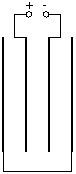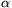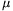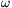Középiskolai Matematikai és Fizikai Lapok
Informatika rovattal
 Már regisztráltál? Új vendég vagy?

# Exercises and problems in Physics January 1998

## New experimental problem:

m. 194. Observe how a candle floating on water with a ballast attached to its bottom burns down. Measure the distance between the ballast and the bottom of the vessel as a function of time. How much does the mass of the extinguished candle differ from the value calculated on the basis of the solution of problem 3062 (published in the December 1997 issue of KóMaL)? Make the measurement with at least two candles of different thicknesses.

## New exercises:

FGy. 3119. The average daily loss of water of a human body is 0.8 kg, evaporated by the organism. How much cheese has to be consumed in order to compensate for the loss of energy due to the evaporation if a human organism can use 70% of the energy provided by the decomposition of aliments. The heat of combustion of cheese is 12,000 kJ/kg, the heat of vaporisation of water is 2440 kJ/kg.

FGy. 3120. How many times does the Earth rotate about its axis with respect to the fixed stars in a year? And the Moon?

FGy. 3121. Before the run-up, a pole-jumper holds his pole of a mass of 4 kg and a length of 6 m horizontally. He exerts a force F1 with one hand at the end of the pole and a force F2 with the other hand at a distance of 0.5 m from the end of the pole. Determine the magnitudes of forces F1 and F2 if their lines of action are perpendicular to the pole. When exercising with another pole, he holds the pole in such a way that it is at an angle of 30o with the horizontal. He pushes the end of the pole horizontally with a force of 28.9 N with one hand and holds it perpendicularly with the other at the suitable point. What is the mass of this pole?

FGy. 3122. What should the total mass of the meteorites hitting the Earth in a year at a speed of 40 km/s be so that in average they cause the same heating as the radiation of the Sun? What mass does this correspond to per second and per square meter?

FGy. 3123. Why do symphonic orchestras tune on the stage of the concert hall and not in the rehearsal room before the performance? List physical reasons that put different instruments (wind-instruments, stringed instruments) out of tune.

## New problems:

FF. 3124. The thread of length l of a yo-yo of mass m and radius R is wound onto the spool of radius r and negligible mass. The yo-yo can be approximated as two cylinders of homogeneous mass distribution. How should we move our hand holding the end of the thread so that the yo-yo rotates in place? How much work do we do while lifting our hand?FF. 3125. Four parallel metal plates of identical areas, facing each other, are connected to each other and to a 220 V DC supply as shown in the picture. The plates are at 2 cm from each other, this distance being small compared to the size of the plates.

a) Determine the magnitude and the direction of the electric field strength in the spaces between the plates.

b) How much energy does this system of capacitors store if the capacitance of a parallel-plate capacitor formed by two of these metal plates placed at 2 cm from each other is known to be 20 pF?

FF. 3126. There is a circular conductor with a radius of 4 cm at some distance from a straight coil with a vertical axis, its plane perpendicular to the axis of the coil and its centre on the axis. The lines of the magnetic induction of the coil are at an angle of 60o with the plane of the circular conductor in the points of the conductor, the magnitude of the induction is 0.08 tesla along the conductor.

a) What is the magnetic force exerted onto the circular conductor when a current of 10 A flows through it?

b) What elastic stress occurs in the conductor with a cross-section of 1 mm2?

FF. 3127. A positron of speed v collides with an electron of negligible speed. Two photons are produced in the process, moving perpendicularly to each other. Determine the energy of the photons. What is the minimum speed of the positron required to produce such photons?

FN. 3128. A small body of mass m is at rest in a sufficiently long tube of radius r at anglewith the horizontal. The coefficient of friction between the tube and the body is(>tg). At what speed and where does the body slide out of the tube when the tube is rotated about its axis at angular velocity?